# Case Study for Plant Layout :: A modern analysis

21 Jan 2009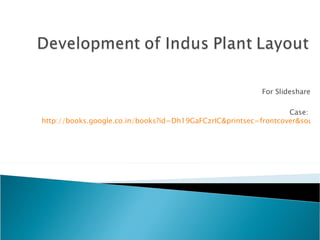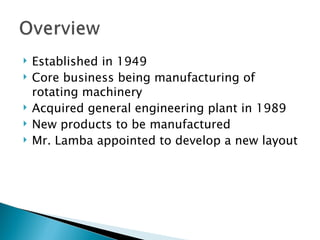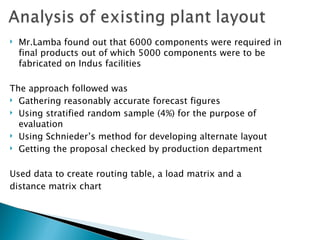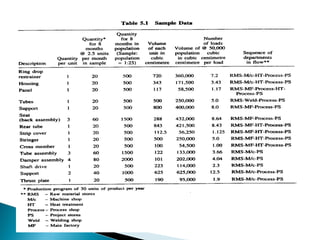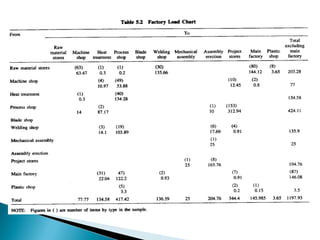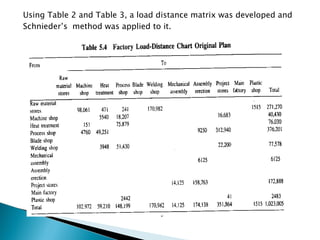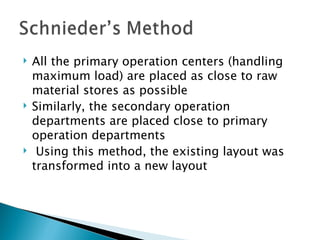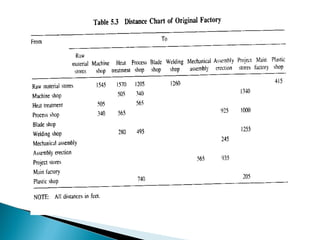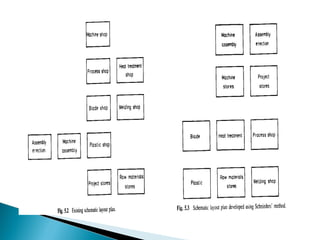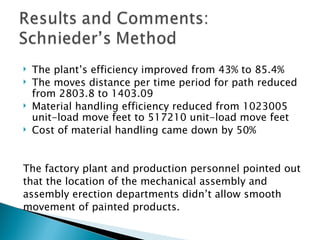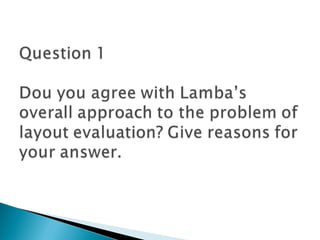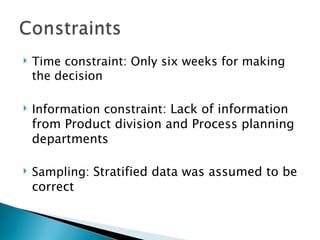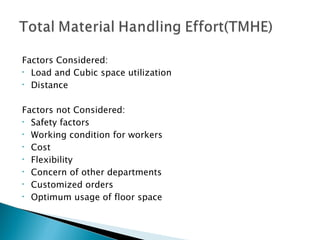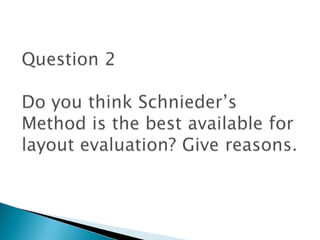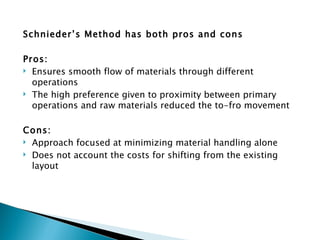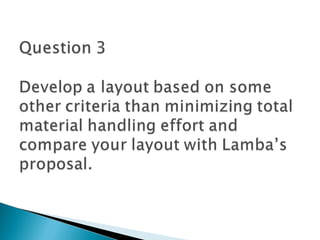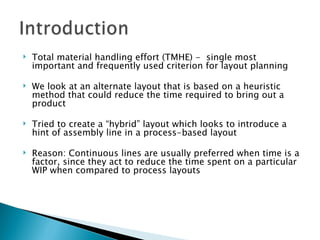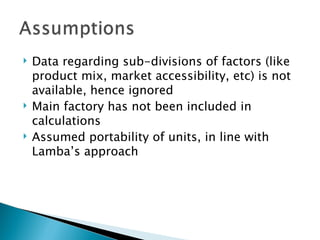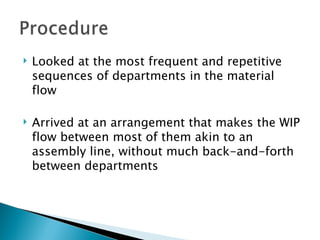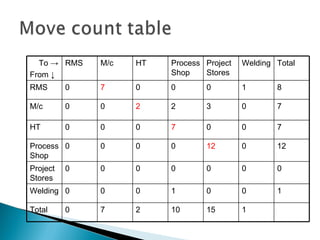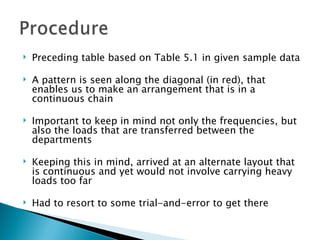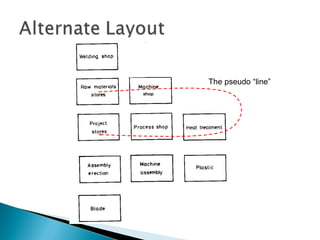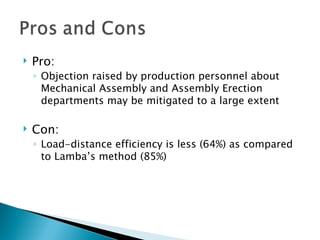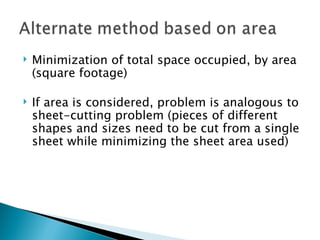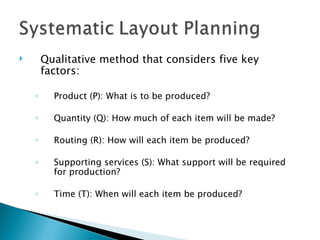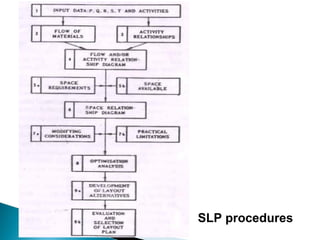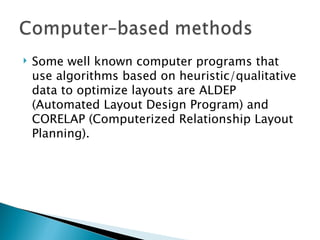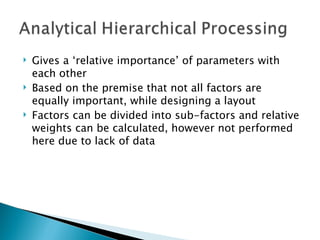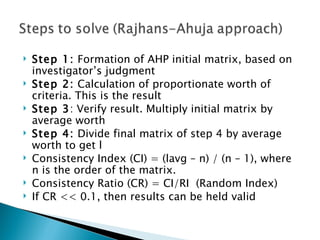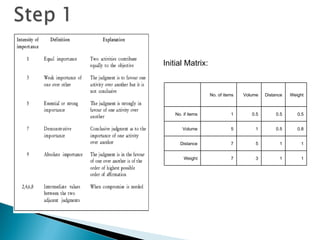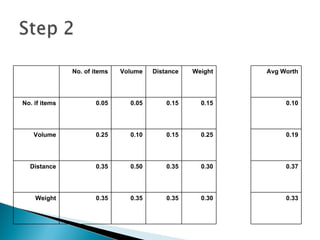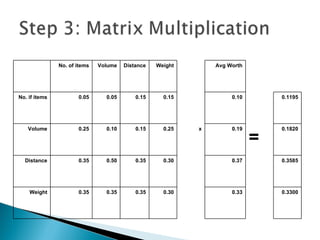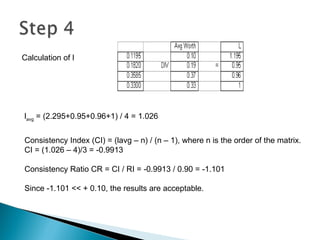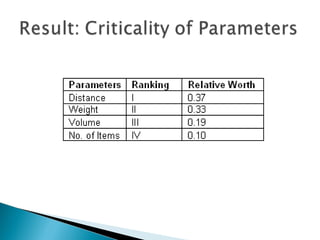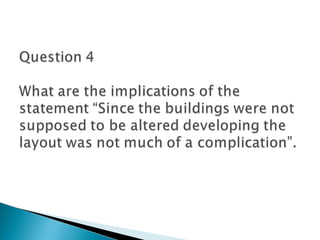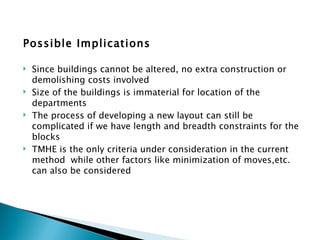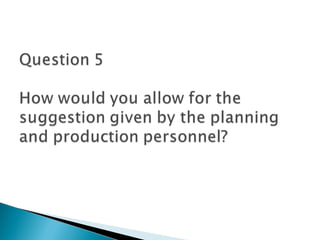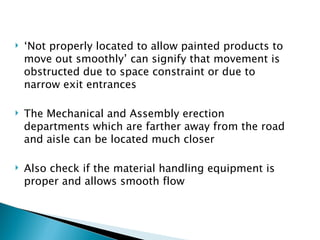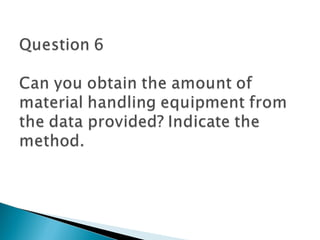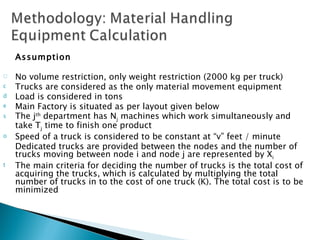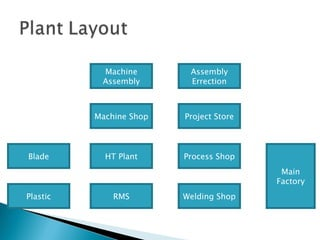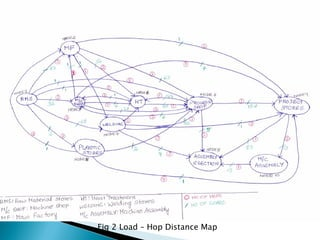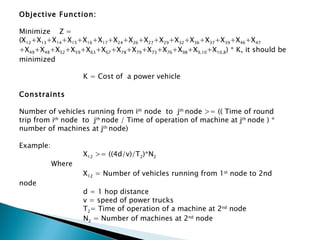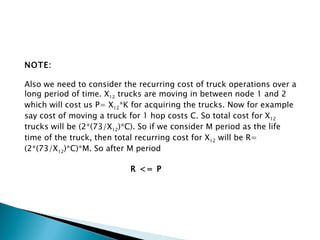1 sur 45

### Case Study for Plant Layout :: A modern analysis

• 2.
• 3.
• 4.
• 5.
• 6.
• 7.
• 8.
• 9.
• 10.
• 11.
• 12.
• 13.
• 14.
• 15.
• 16.
• 17.
• 18.
• 19.
• 20. To -> From ↓ RMS M/c HT Process Shop Project Stores Welding Total RMS 0 7 0 0 0 1 8 M/c 0 0 2 2 3 0 7 HT 0 0 0 7 0 0 7 Process Shop 0 0 0 0 12 0 12 Project Stores 0 0 0 0 0 0 0 Welding 0 0 0 1 0 0 1 Total 0 7 2 10 15 1
• 21.
• 23.
• 24.
• 25.
• 27.
• 28.
• 29.
• 30. Initial Matrix:   No. of items Volume Distance Weight No. if items 1 0.5 0.5 0.5 Volume 5 1 0.5 0.8 Distance 7 5 1 1 Weight 7 3 1 1
• 31.   No. of items Volume Distance Weight Avg Worth No. if items 0.05 0.05 0.15 0.15 0.10 Volume 0.25 0.10 0.15 0.25 0.19 Distance 0.35 0.50 0.35 0.30 0.37 Weight 0.35 0.35 0.35 0.30 0.33
• 32.   No. of items Volume Distance Weight Avg Worth No. if items 0.05 0.05 0.15 0.15 0.10   0.1195 Volume 0.25 0.10 0.15 0.25 x 0.19 =   0.1820 Distance 0.35 0.50 0.35 0.30 0.37   0.3585 Weight 0.35 0.35 0.35 0.30 0.33   0.3300
• 33. l avg = (2.295+0.95+0.96+1) / 4 = 1.026 Consistency Index (CI) = (lavg – n) / (n – 1), where n is the order of the matrix. CI = (1.026 – 4)/3 = -0.9913 Consistency Ratio CR = CI / RI = -0.9913 / 0.90 = -1.101 Since -1.101 << + 0.10, the results are acceptable. Calculation of l
• 34.
• 35.
• 36.
• 37.
• 38.
• 39.
• 40.
• 41. Machine Assembly Machine Shop Welding Shop Process Shop Project Store Assembly Errection HT Plant RMS Blade Plastic Main Factory
• 42. Fig 2 Load – Hop Distance Map
• 43. Objective Function: Minimize Z = (X 12 +X 13 +X 14 +X 15 +X 16 +X 17 +X 24 +X 26 +X 27 +X 29 +X 32 +X 36 +X 37 +X 39 +X 46 +X 47 +X 49 +X 48 +X 52 +X 59 +X 63 +X 67 +X 78 +X 79 +X 73 +X 76 +X 98 +X 9,10 +X 10,8 ) * K, it should be minimized   K = Cost of a power vehicle Constraints   Number of vehicles running from i th node to j th node >= (( Time of round trip from i th node to j th node / Time of operation of machine at j th node ) * number of machines at j th node)   Example:  X 12 >= ((4d/v)/T 2 )*N 2   Where X 12 = Number of vehicles running from 1 st node to 2nd node d = 1 hop distance v = speed of power trucks T 2 = Time of operation of a machine at 2 nd node N 2 = Number of machines at 2 nd node
• 44. NOTE: Also we need to consider the recurring cost of truck operations over a long period of time. X 12 trucks are moving in between node 1 and 2 which will cost us P= X 12 *K for acquiring the trucks. Now for example say cost of moving a truck for 1 hop costs C. So total cost for X 12 trucks will be (2*(73/X 12 )*C). So if we consider M period as the life time of the truck, then total recurring cost for X 12 will be R= (2*(73/X 12 )*C)*M. So after M period   R <= P